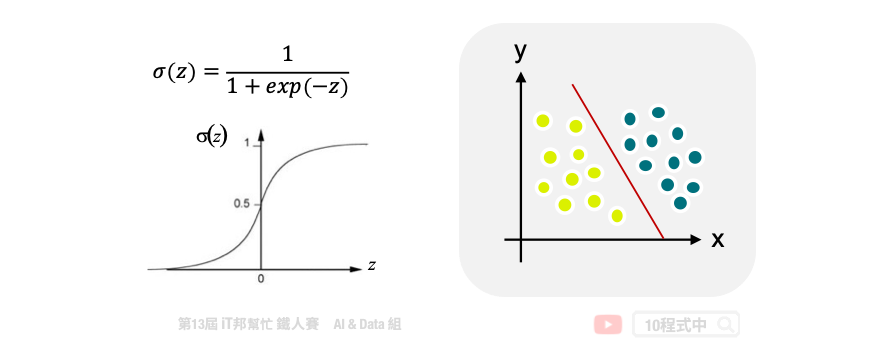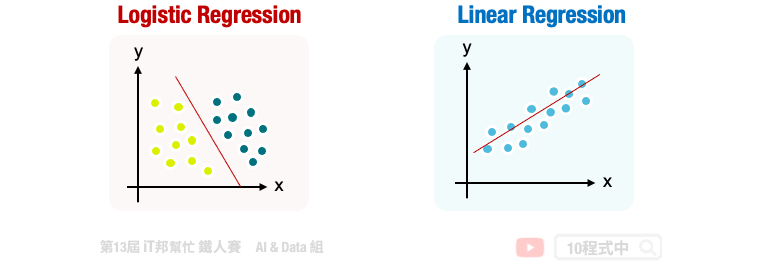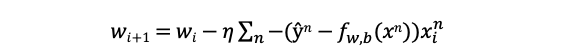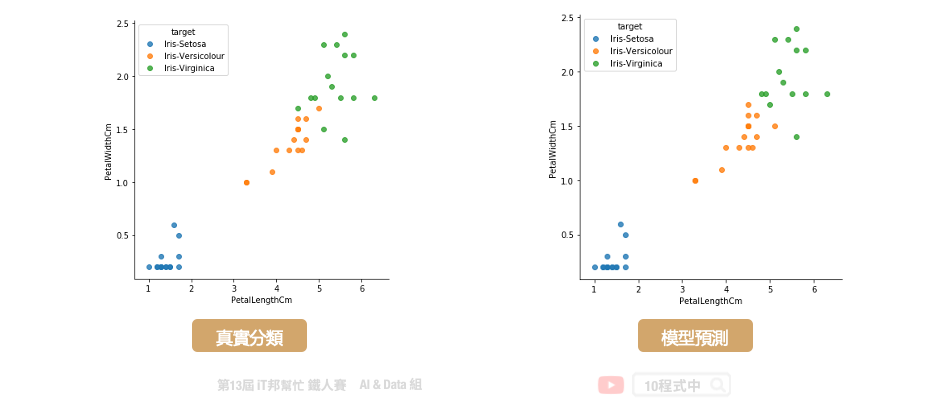DAY 9
0
AI & Data

# 邏輯迴歸 (Logistic Regression)

## 今日學習目標

• 認識邏輯迴歸
• 線性分類器
• 邏輯迴歸學習機制
• 比較線性迴歸與邏輯迴歸
• 多元分類邏輯迴歸
• 邏輯迴歸程式手把手
• 使用邏輯迴歸建立鳶尾花朵分類器

## 認識邏輯迴歸## 線性迴歸與邏輯迴歸## 邏輯迴歸學習機制## 多元分類邏輯迴歸 (Multinomial Logistic Regression)

• one-vs-rest(OvR): 訓練時把某個類別的資料歸為一類，其他剩餘的資料歸為另一類做邏輯迴歸，因此若有 k 個類別的資料會有 k 個二元分類器。假如有三個類別 A、B、C，首先抽取 A 類別的資料做為正集，B、C 類別資料做為負集; B 類別的資料作為正集，A、C 類別類別資料做為負集; C 類別的資料作為正集，A、B 類別類別資料做為負集。透過這三組訓練集分別進行訓練，然後的得到三個分類器 f1(x)、f2(x)、f3(x)。預測的時候就是把資料丟進三個分類器，查看哪個分類器預測的分數最高就決定該類別。
• many-vs-many(MvM): 與 OvR 差別在於訓練時每次只會挑兩個類別訓練一個分類器，因此 k 個類別的資料就需要 k(k-1)/2 個二元分類器。假如有三個類別 A、B、C，因此我們會有三組二元分類器分別有 (A、B)、(A、C) 與 (B、C)。訓練完成後當有新資料要預測時，把資料分別對三個二元分類器進行預測，最終多數決的方式得到預測結果。

## 邏輯迴歸 (分類器)

Parameters:

• penalty: 正規化l1/l2，防止模型過度擬合。
• C: 數值越大對 weight 的控制力越弱，預設為1。
• n_init: 預設為10次隨機初始化，選擇效果最好的一種來作為模型。
• solver: 優化器的選擇。newton-cg,lbfgs,liblinear,sag,saga。預設為liblinear。
• multi_class: 選擇分類方式，ovr就是one-vs-rest(OvR)，而multinomial就是many-vs-many(MvM)。預設為 auto，故模型訓練中會取一個最好的結果。
• max_iter: 迭代次數，預設為100代。
• class_weight: 若遇資料不平衡問題可以設定balance，預設=None。
• random_state: 亂數種子僅在solver=sag/liblinear時有用。

Attributes:

• coef_: 取得斜率。
• intercept_: 取得截距。

Methods:

• fit: 放入X、y進行模型擬合。
• predict: 預測並回傳預測類別。
• predict_proba: 預測每個類別的機率值。
• score: 預測成功的比例。
``````from sklearn.linear_model import LogisticRegression

# 建立Logistic模型
logisticModel = LogisticRegression(random_state=0)
# 使用訓練資料訓練模型
logisticModel.fit(X_train, y_train)
# 使用訓練資料預測分類
predicted = logisticModel.predict(X_train)
``````

### 使用Score評估模型

``````# 預測成功的比例
print('訓練集: ',logisticModel.score(X_train,y_train))
print('測試集: ',logisticModel.score(X_test,y_test))
``````

``````訓練集:  0.9714285714285714

``````